## 7.1 The Problem of Overfitting

Regularization 正则化
Normalization 归一化

quiz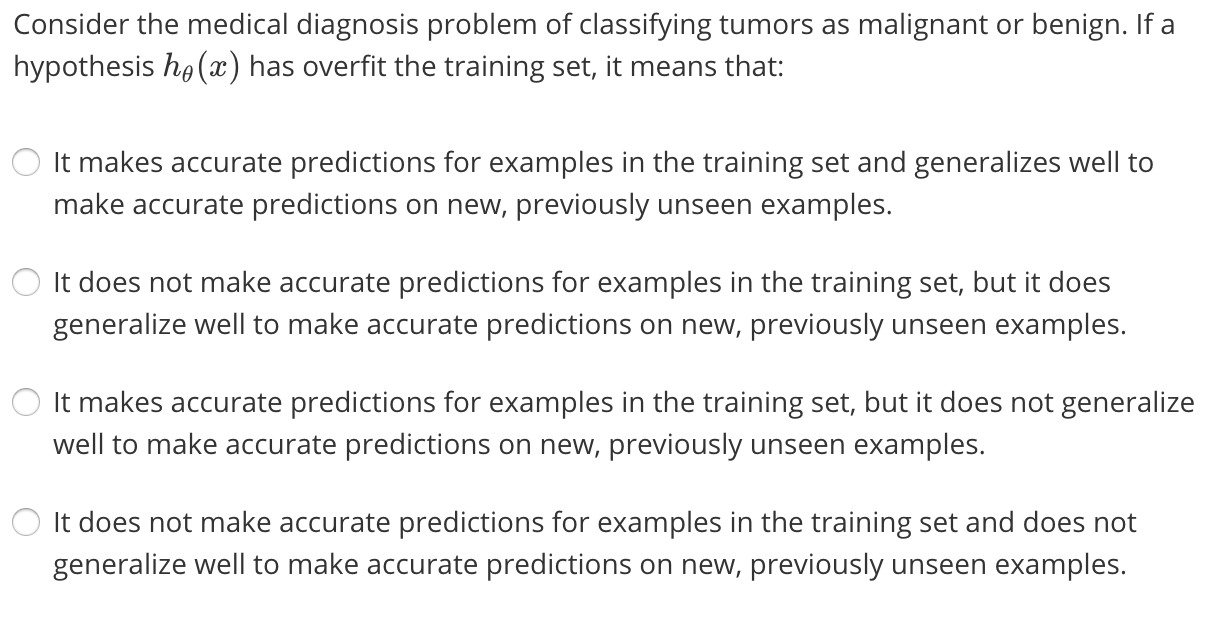1. 减少 feature 数量
• 手动选择
• 设计算法自动选择feature（Model selection algorithm后面会讲）
2. 正则化 Regularization
• 保留所有 feature，但是减少每个 $\theta_j$的 magnitude 值
• 对于有很多 feature，每一个也都有一点点作用的情况很管用

## 7.2 Cost Function

penalize 惩罚
prone 倾向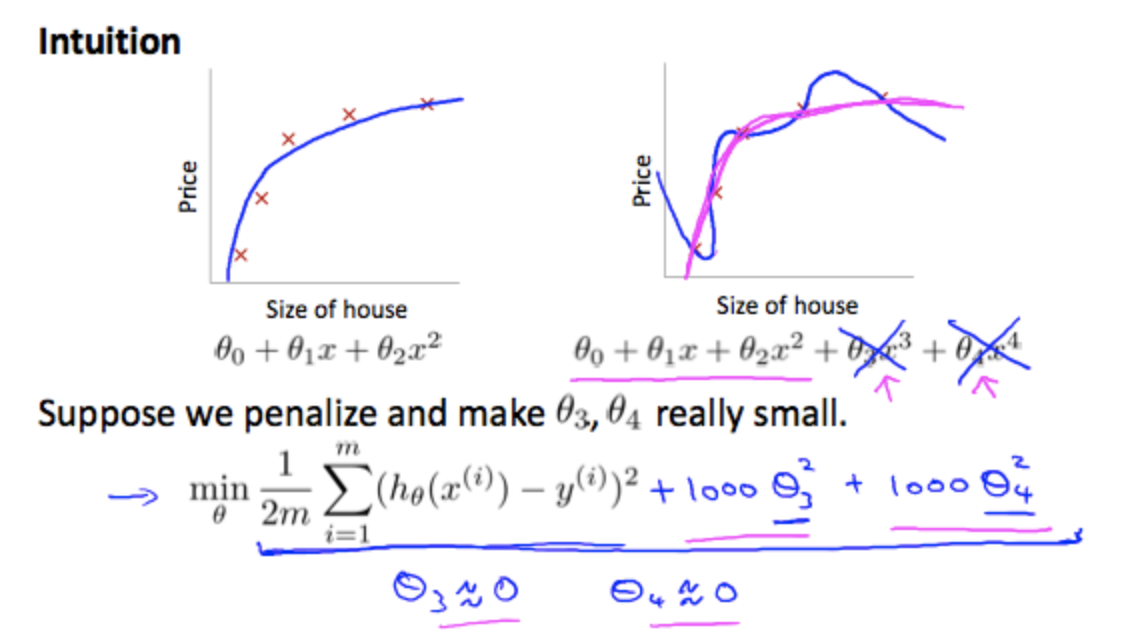quiz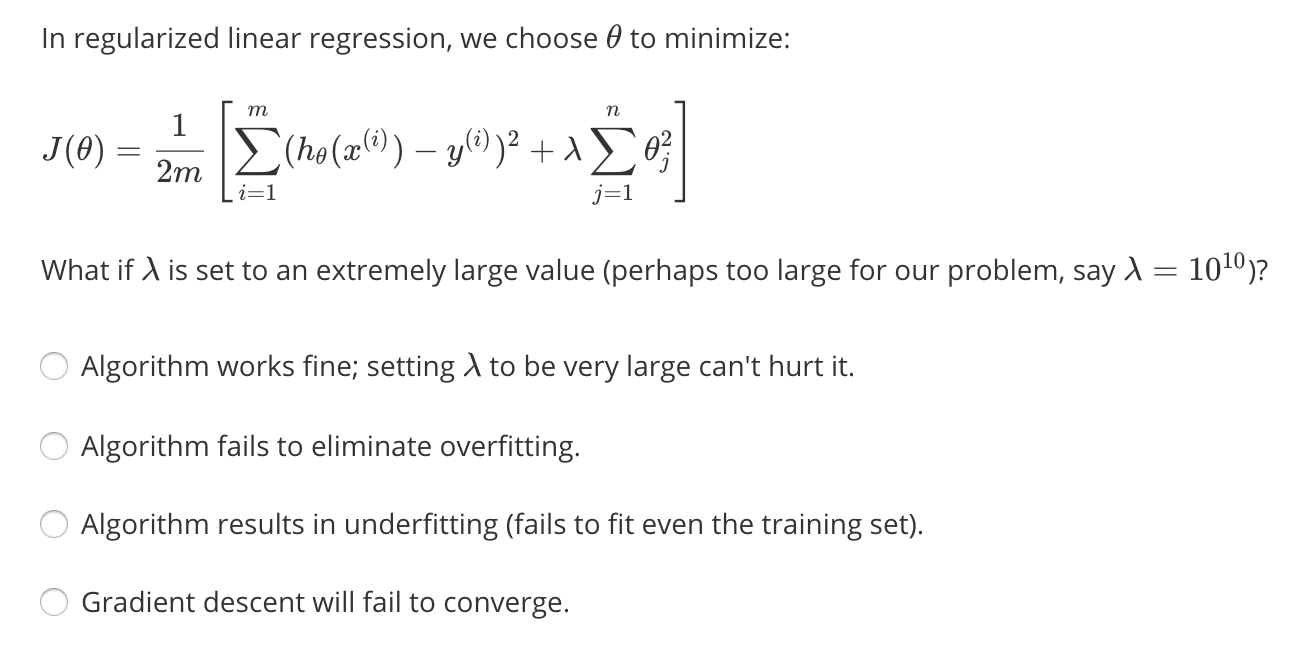Answe: 第三项 ，会出现欠拟合了，$\lambda$ 要大但是也不能太大，太大的话会产生太多惩罚，而$\theta_0$的重要性就会大大增加，曲线会逼近于直线。

## 7.3 Regularized Linear Regression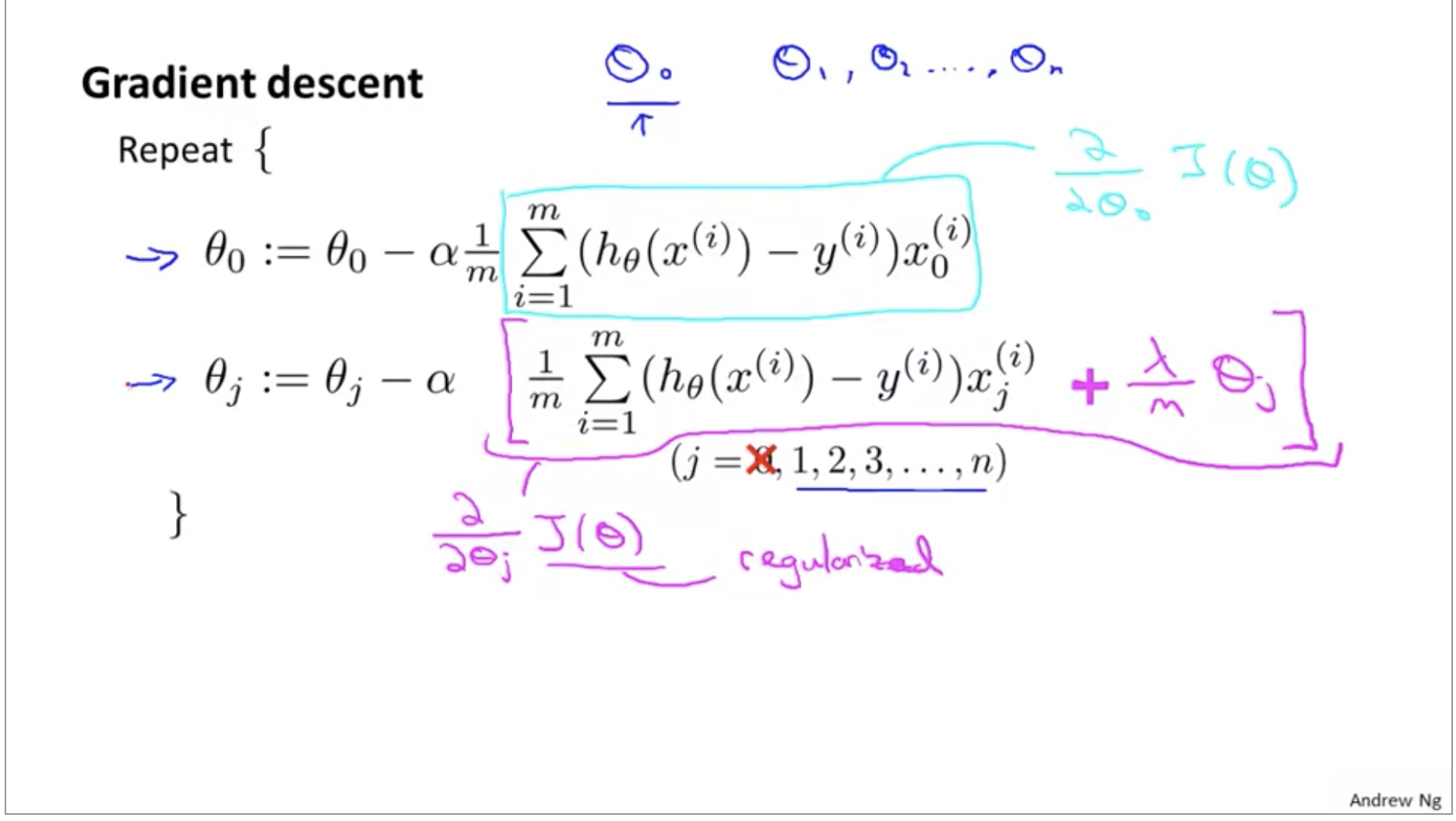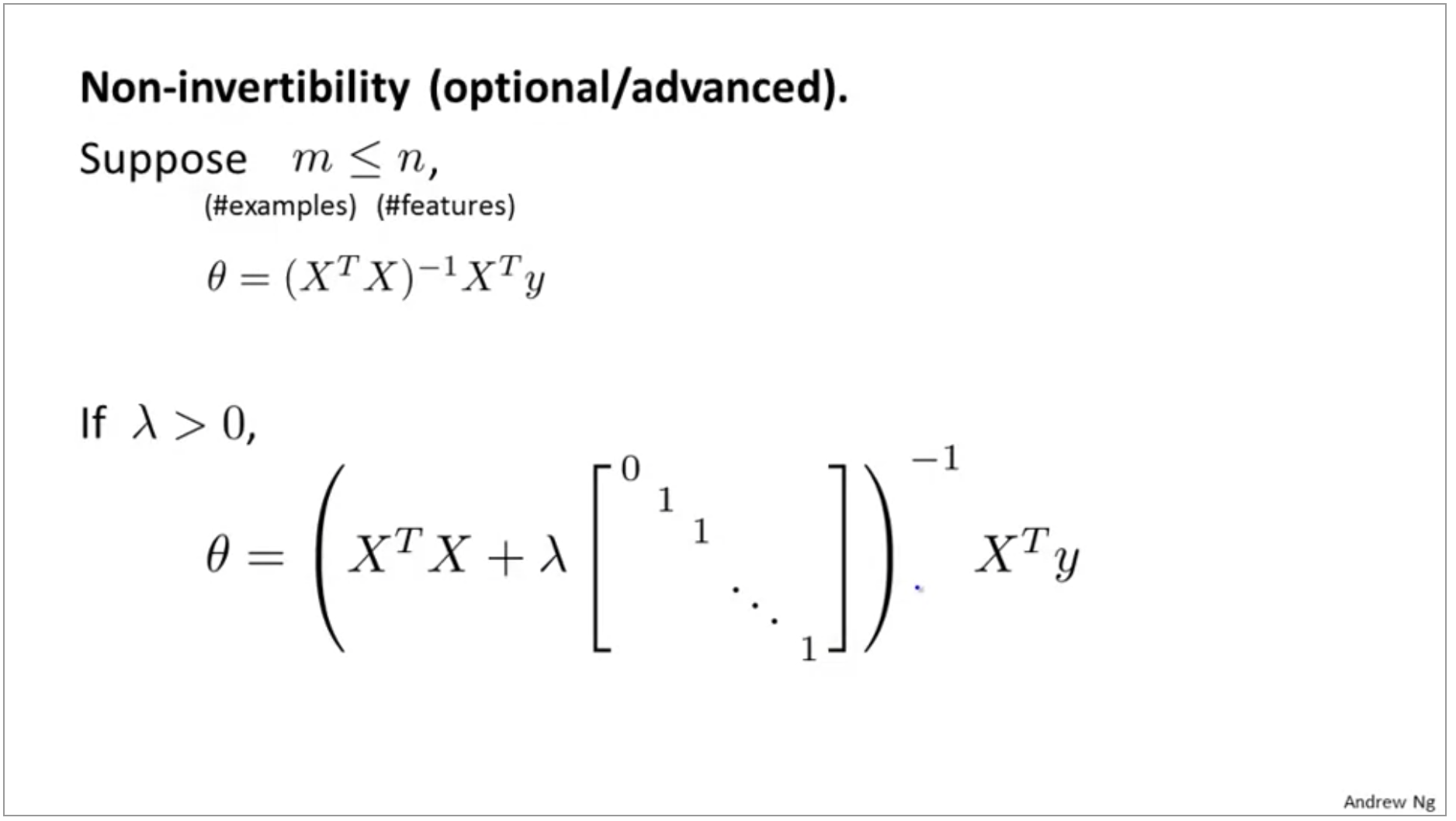## 7.4 Regularized Logistic Regression

quiz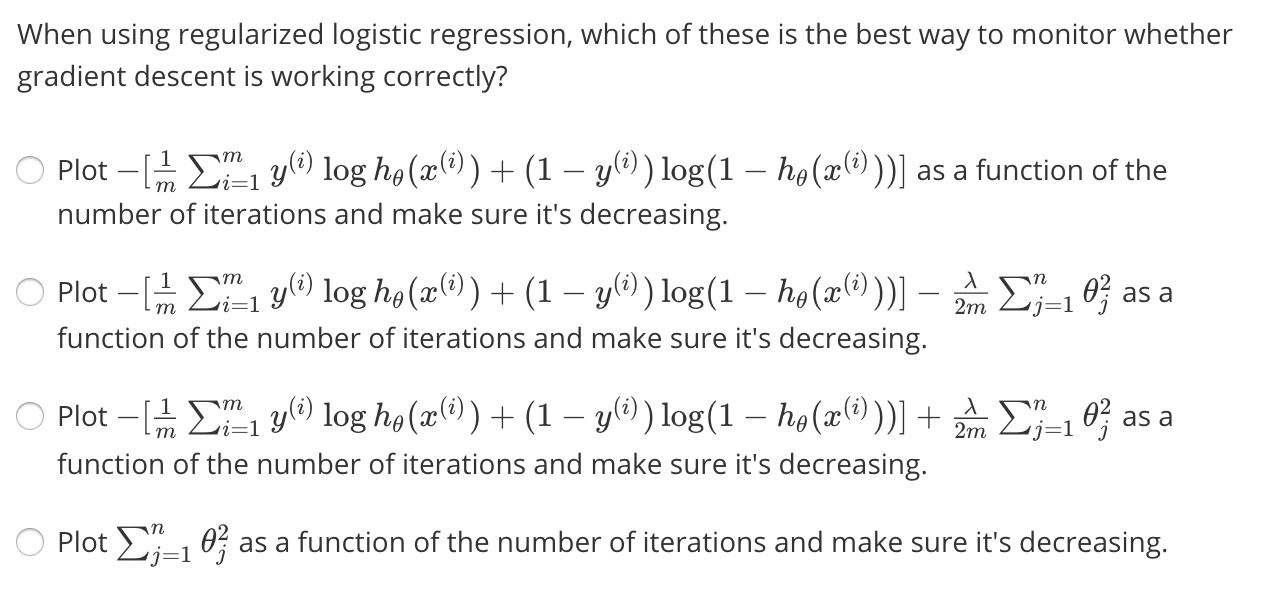Answe:第三项

## Test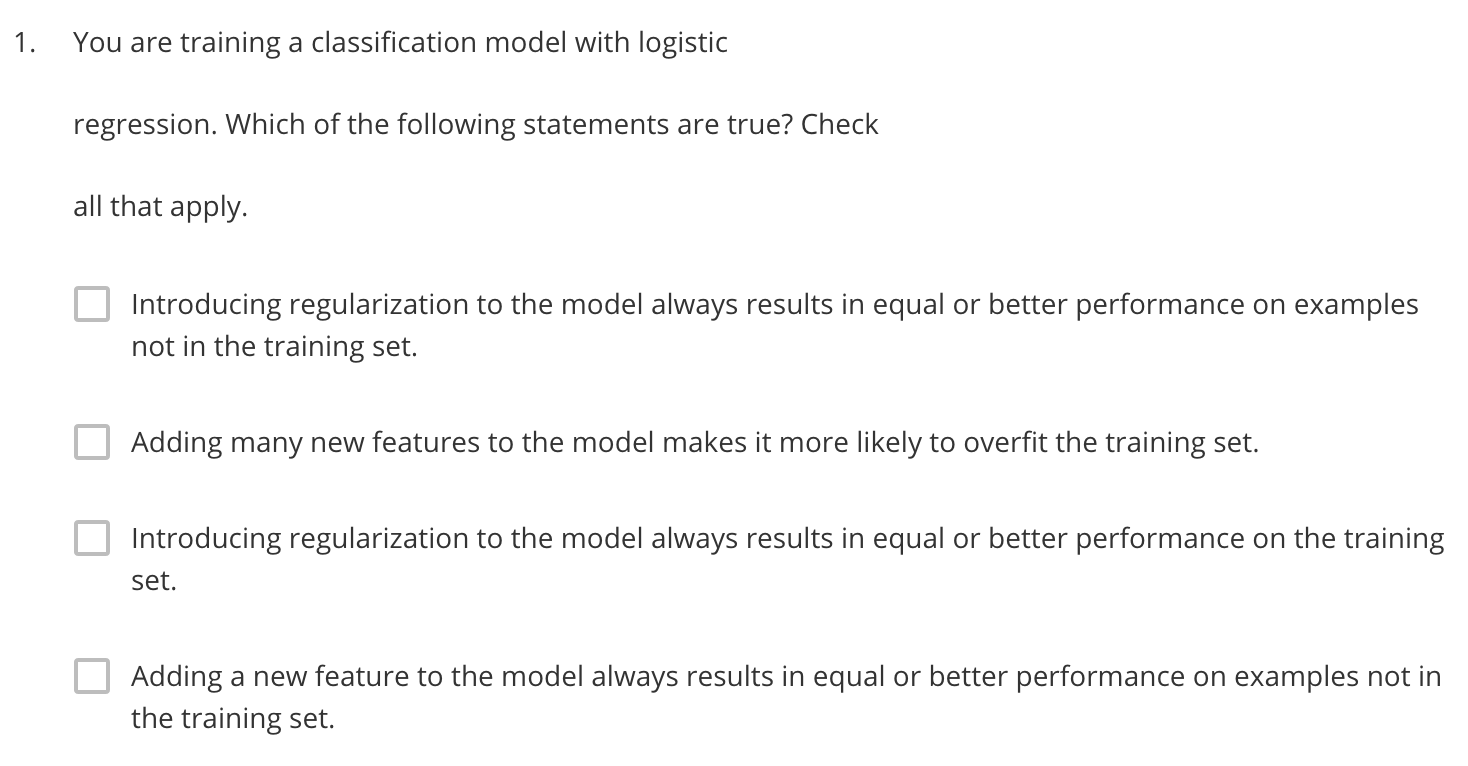Answe:第二项，第一项如果$\lambda$太大也有可能造成欠拟合，而对于训练集一般是效果会差一点。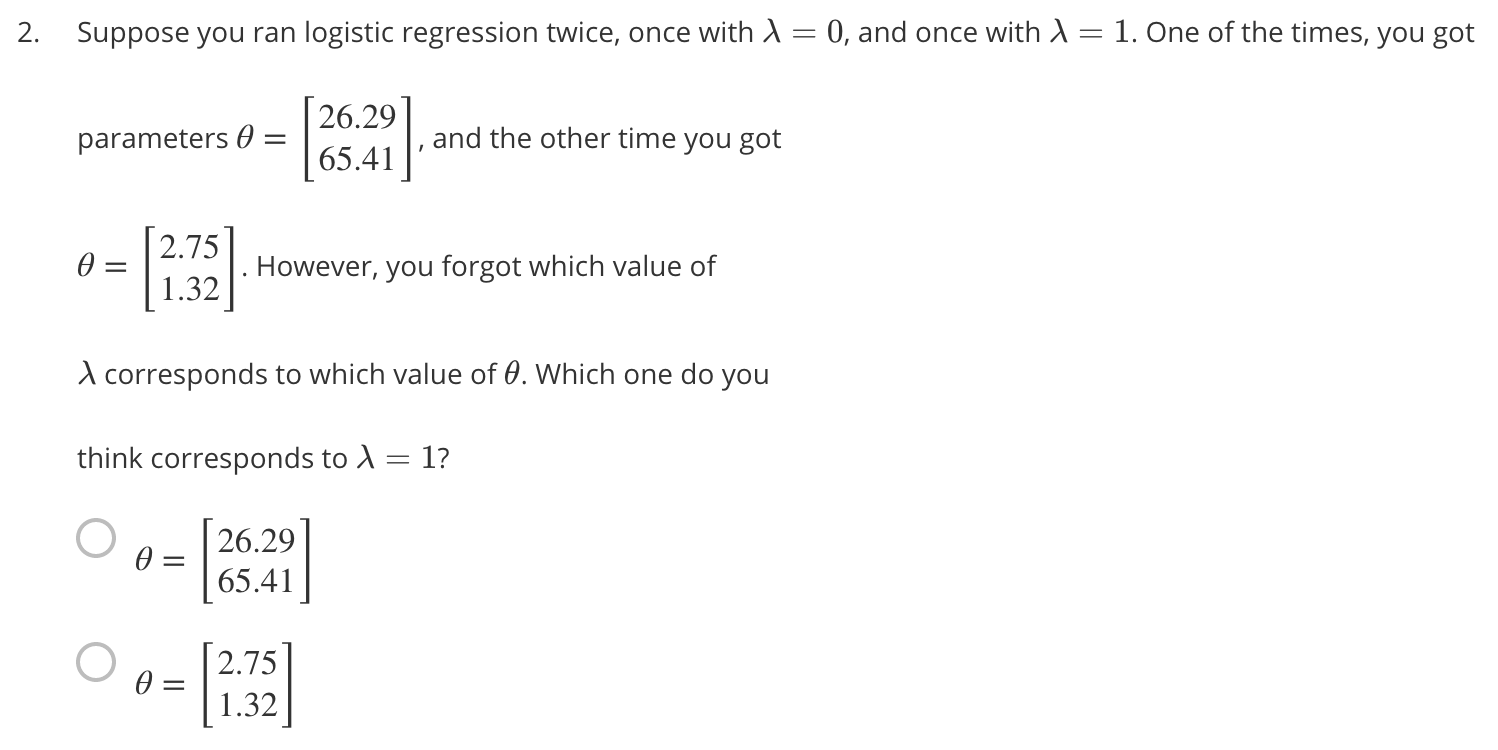Answe:第二项 $\lambda=1$的时候$\theta_0$的重要性会上升。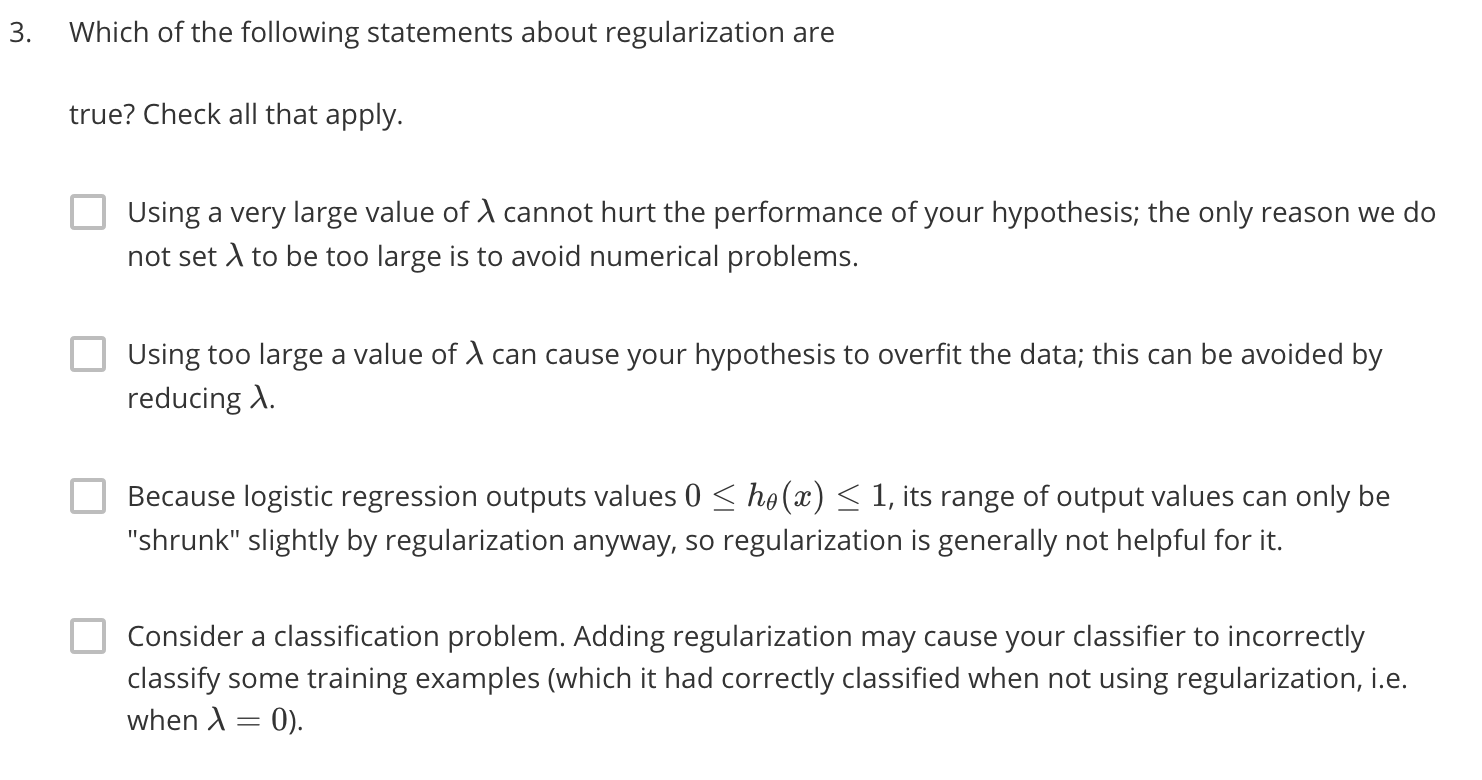Answe:第四项 过大的$\lambda$会造成欠拟合而不是过拟合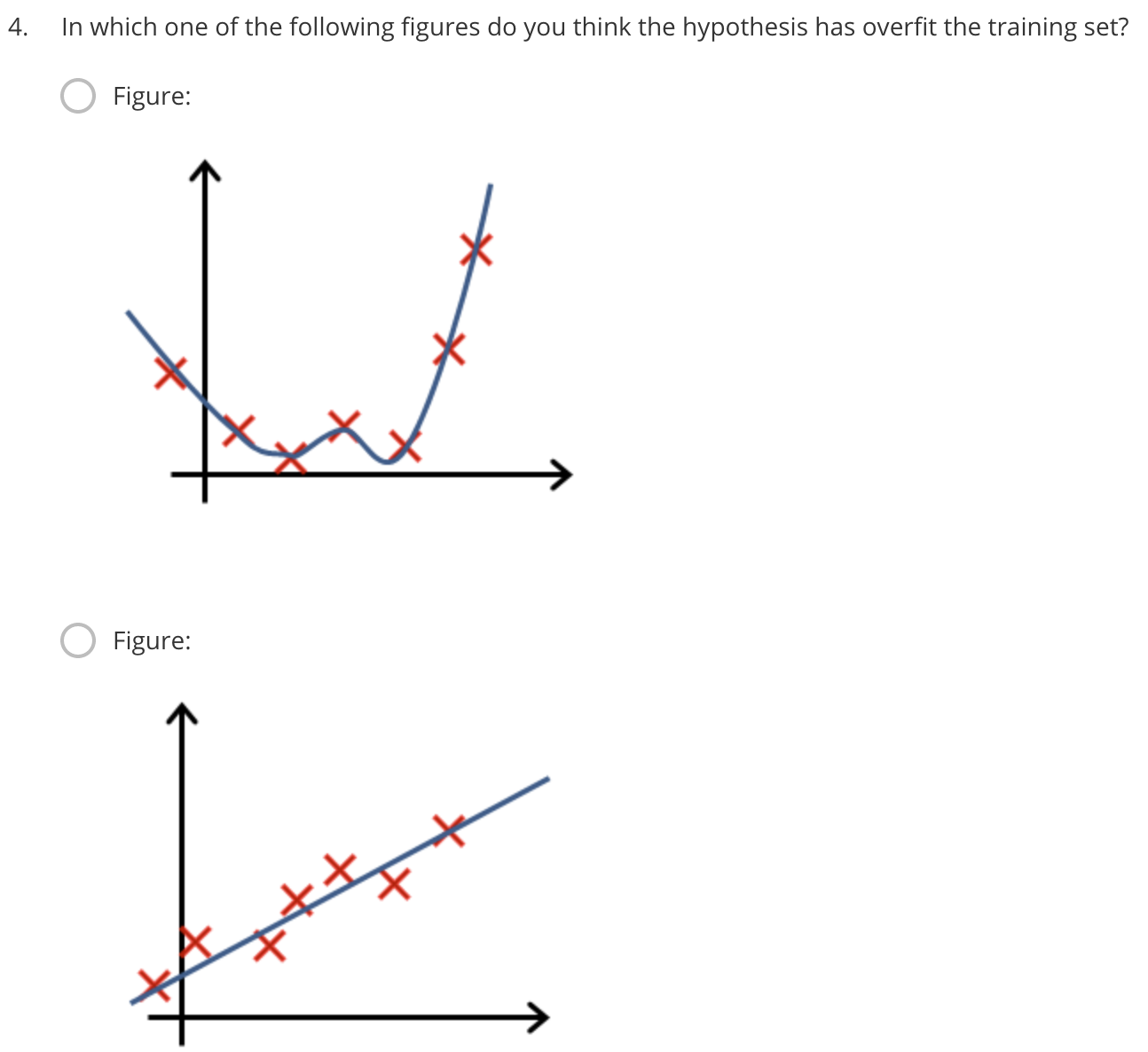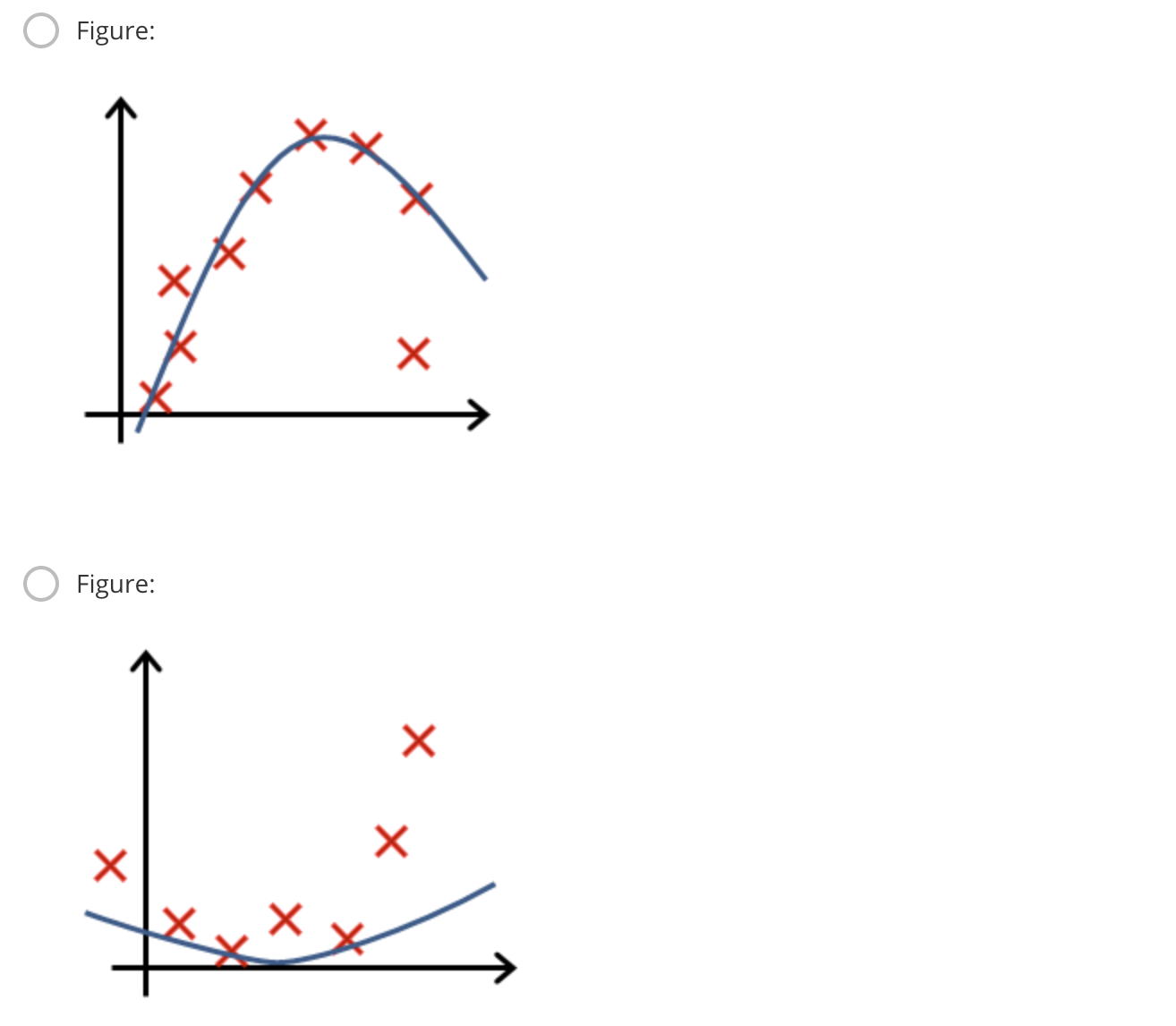Answe:第一项 找过拟合的显然第一个。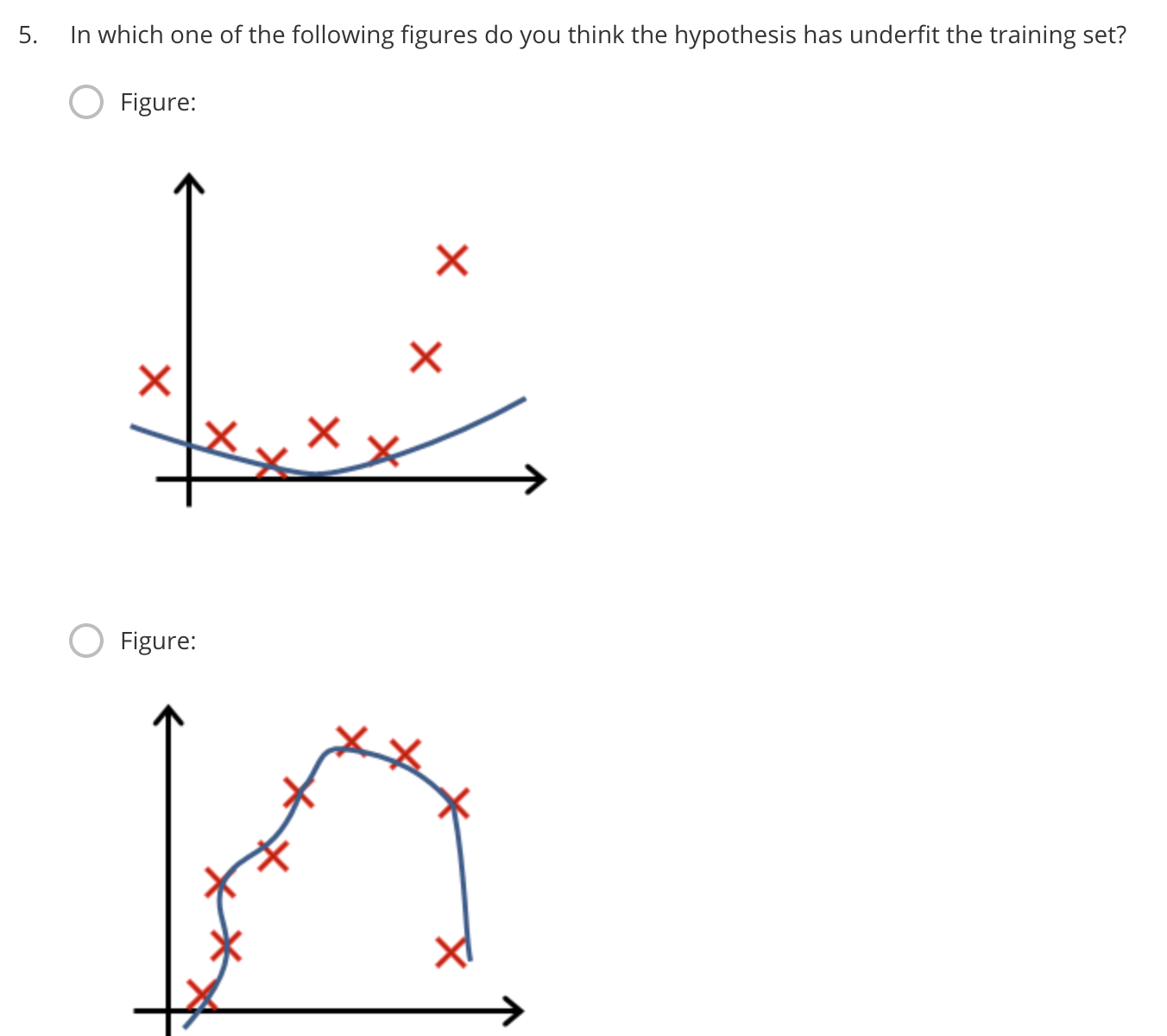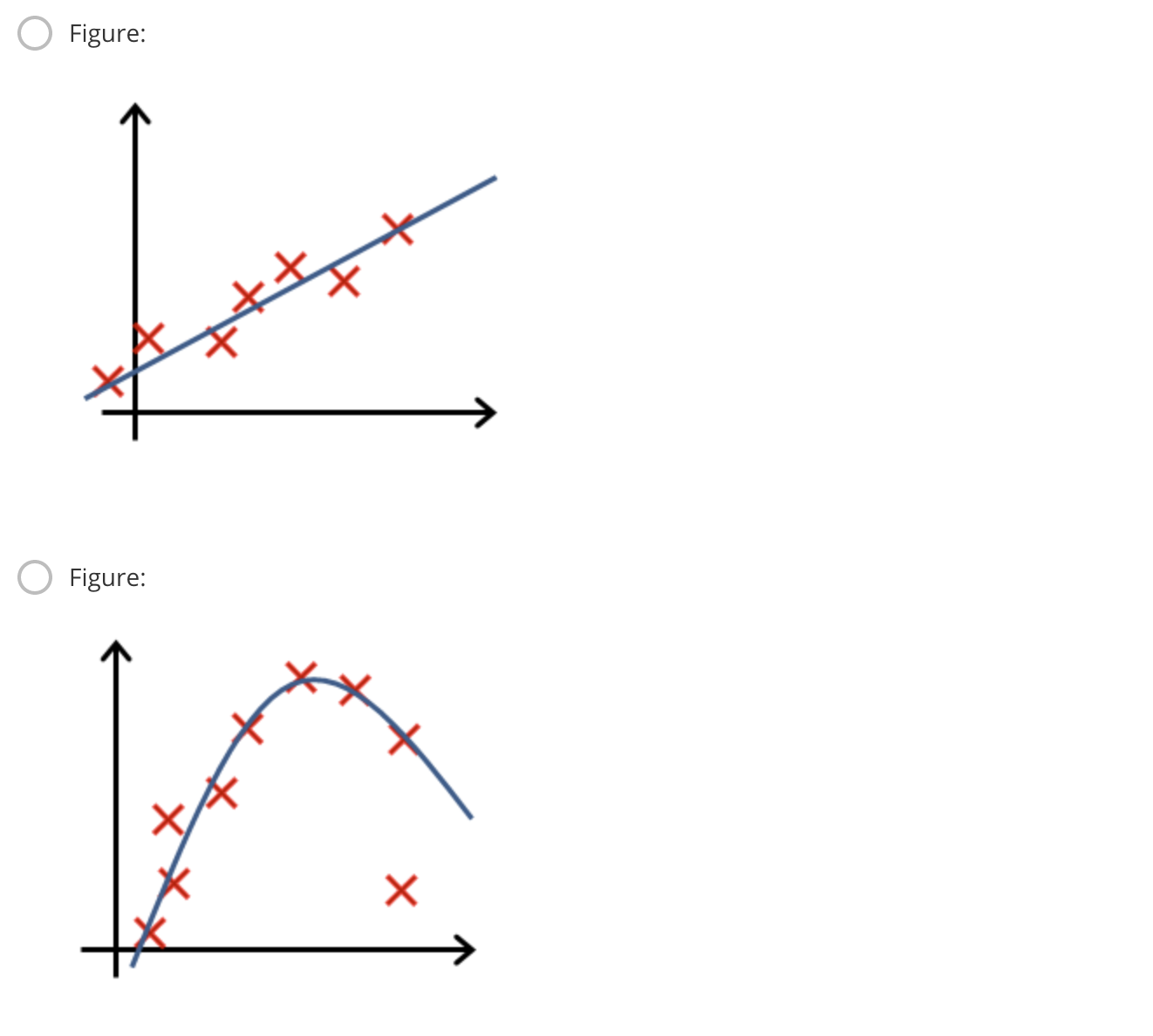Answe:第一项 找欠拟合的第一个